# Open intervals

Open intervals A = (x-2; 2x-1) and B = (3x-4; 4) are given. Find the largest real number for which A ⊂ B applies.

x =  2.5

### Step-by-step explanation: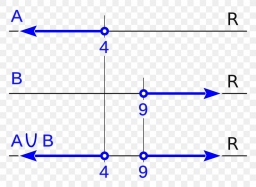Did you find an error or inaccuracy? Feel free to write us. Thank you!## Related math problems and questions:

• Intersect and conjuctionLet U={1,2,3,4,5,6} A={1,3,5} B={2,4,6} C={3,6} Find the following. 1. )AUB 2. )A'UB'
• Median or middle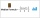The number of hours of television watched per day by a sample of 28 people is given below: 4, 1, 5, 5, 2, 5, 4, 4, 2, 3, 6, 8, 3, 5, 2, 0, 3, 5, 9, 4, 5, 2, 1, 3, 4, 7, 2, 9 What is the median value?
• Three digits number 2Find the number of all three-digit positive integers that can be put together from digits 1,2,3,4 and which are subject to the same time has the following conditions: on one positions is one of the numbers 1,3,4, on the place of hundreds 4 or 2.
• The HotelThe Holiday Hotel has the same number of rooms on each floor. Rooms are numbered with natural numerals sequentially from the first floor, no number is omitted, and each room has a different number. Three tourists arrived at the hotel. The first one was in
• Is complexAre these numbers 2i, 4i, 2i + 1, 8i, 2i + 3, 4 + 7i, 8i, 8i + 4, 5i, 6i, 3i complex?
• Francesca 2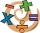Francesca applied the steps below to find the product of (-1.2)(-9.4). Step 1: (-1.2)(-9.4) = (-9.4)(-1.2) Step 2:                   = (-9.4)(-1) + (-9.4)(-0.2) Step 3:                   = (9.4) + (1.88) Step 4:                   = 11.28 Which step shows
• Four-digit number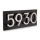For a four-digit number abcd, ab: bc = 1: 3 and bc: cd = 2: 1 (ab, bc and cd are two-digit numbers from digits a, b, c, d). Find this number(s).
• Three digits numberFrom the numbers 1, 2, 3, 4, 5 create three-digit numbers that digits not repeat and number is divisible by 2. How many numbers are there?
• Evaluate mixed expressionsWhich of the following is equal to 4 and 2 over 3 divided by 3 and 1 over 2? A. 4 and 2 over 3 times 3 and 2 over 1 B. 14 over 3 times 2 over 7 C. 14 over 3 times 7 over 2 D. 42 over 3 times 2 over 31
• CoordinatesDetermine the coordinates of the vertices and the content of the parallelogram, the two sides of which lie on the lines 8x + 3y + 1 = 0, 2x + y-1 = 0 and the diagonal on the line 3x + 2y + 3 = 0
• Percent to interval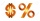76% is between which of the following two numbers. 1) 1/5 & 1/4. 2) 1/3 & 1/2 3) 2/3 & 7/10 4) 3/4 & 5/6
• Vector perpendicularFind the vector a = (2, y, z) so that a⊥ b and a ⊥ c where b = (-1, 4, 2) and c = (3, -3, -1)
• Air draftThe numbers 1,2,3,4,5 are written on five tickets on the table. Air draft randomly shuffled the tickets and composed a 5-digit number from them. What is the probability that he passed: and, the largest possible number b, the smallest possible number c, a
• Find the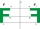Find the image A´ of point A [1,2] in axial symmetry with the axis p: x = -1 + 3t, y = -2 + t (t = are real number)Which of the points belong function f:y= 2x2- 3x + 1 : A(-2, 15) B (3,10) C (1,4)Cat's school use a special numerical axis. The distance between the numbers 1 and 2 is 1 cm, the distance between the numbers 2 and 3 is 3 cm, between the numbers 3 and 4 is 5 cm and so on, the distance between the next pair of natural numbers is always iRefer to the sets below: U = {1,2,3,. .. ,10} A = {1,2,3,4,5} B = {2,4,6,7,8} C = {4,5,6,8,10} A ∩ B ∩ C = ___